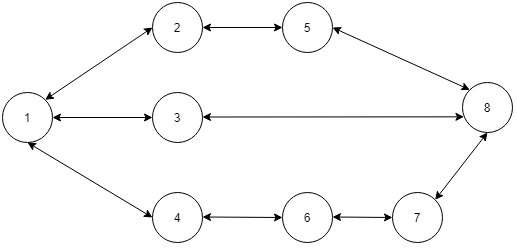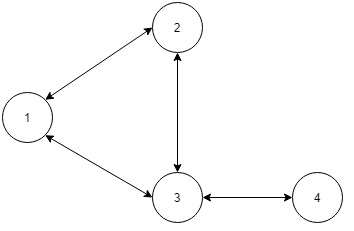3

# Shortest path in an unweighted graph

Difficulty: EASY
Avg. time to solve
25 min
Success Rate
70%

Problem Statement
Suggest Edit

#### A path between house S to house T is defined as a sequence of vertices from S to T. Where starting house is S and ending house is T and there is a road connecting two consecutive houses. Basically, the path looks like this: (S , h1 , h2 , h3 , ... T). you have to find the shortest path from S to T.

``````For example, In the below map of ninja land let say you want to go from
S=1 to T=8, the shortest path is (1, 3, 8). You can also go from S=1
to T=8  via (1, 2, 5, 8)  or (1, 4, 6, 7, 8) but these paths are not shortest.
``````##### Input Format :
``````The first line of input will have a single positive integer T , of test cases.

The first line of each test case has two positive integers N and M denoting the number of houses and the number of roads in the ninja land.

The second line contains two integers S and T denoting the starting and ending house in the path.

Next M lines each contain two integers X and Y. Which denotes a road between house X and house Y.
``````
##### Output Format :
``````You have to return a vector of nodes denoting the shortest path starting from S and ending in T.

If there is more than one shortest path you can return any one of them.
``````
##### Note :
``````You do not need to print anything, it has already been taken care of. Just implement the given function.
``````
##### Constraints :
``````1 <= T <= 100
2 <= N <= 10^3
1 <= M <= min( N*(N-1)/2 , 1000 )
1 <= S,T <= N

Time Limit: 1 sec
``````
##### Sample input 1 :
``````1
4 4
1 4
1 2
2 3
3 4
1 3
``````
##### Sample Output 1 :
``````( 1 , 3 , 4 )
``````
##### Explanation of Sample input 1 :
``````In the above graph there are two ways to go from 1 to 4 ,
( 1 , 2 , 3 , 4 ) and ( 1 , 3 , 4 ) but the second path is the shortest path.
``````##### Sample input 2 :
``````1
8 9
1 8
1 2
1 3
1 4
2 5
5 8
3 8
4 6
6 7
7 8
``````
##### Sample output 2 :
``````( 1 , 3 , 8 )
``````Want to solve this problem? Login now to get access to solve the problems